0
1
01. 云栖社区>
2. paperweekly>
3. 博客>
4. 正文

揭开迷雾，来一顿美味的「Capsule」盛宴 | 附代码实现 + 全新实验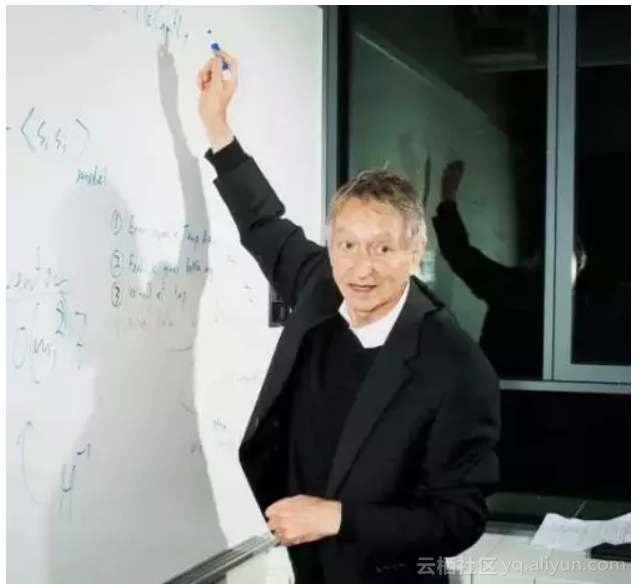• Capsule 是什么？
• Capsule 为什么要这样做？
• Capsule 真的好吗？
• 我觉得 Capsule 怎样？
• 若干小菜

Capsule 的论文已经放出几个月了，网上已经有很多大佬进行解读，也有大佬开源实现了 CapsuleNet，这些内容都加速了我对 Capsule 的理解。然而，我觉得美中不足的是，网上多数的解读，都只是在论文的翻译上粉饰了一点文字，并没有对 Capsule 的原理进行解读。

Capsule 盛宴

Capsule 的革命在于：它提出了一种新的“vector in vector out”的传递方案，并且这种方案在很大程度上是可解释的。

Capsule 就是针对着这个“层层递进”的目标来设计的，但坦白说，Capsule 论文的文笔真的不敢恭维，因此本文尽量不与论文中的符号相同，以免读者再次云里雾里。让我们来看个图。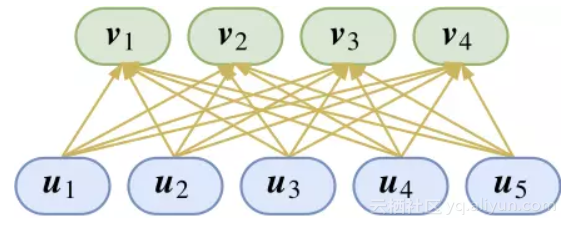Hinton 的理解是：每一个胶囊表示一个属性，而胶囊的向量则表示这个属性的“标架”。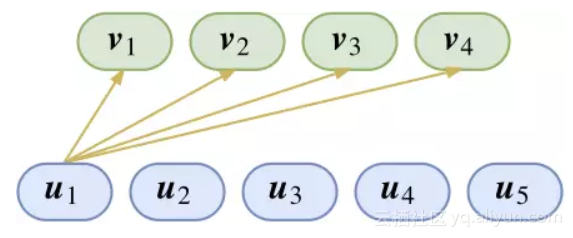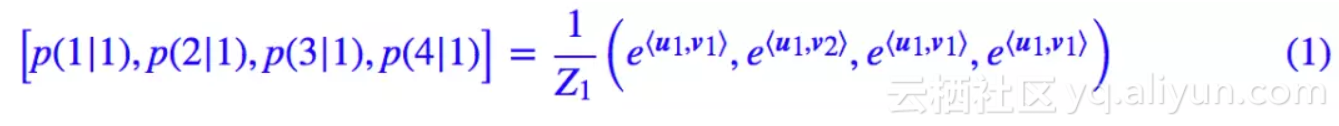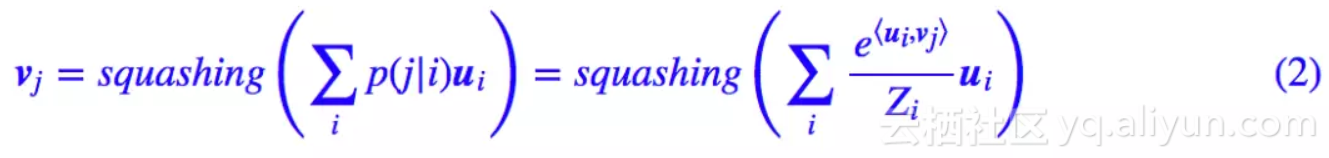squash 在英文中也有浓缩果汁之意，我们就当它是一杯果汁品尝吧。这杯果汁的出现，是因为 Hinton 希望 Capsule 能有的一个性质是：胶囊的模长能够代表这个特征的概率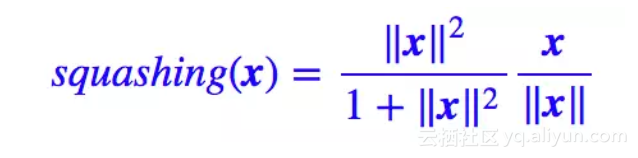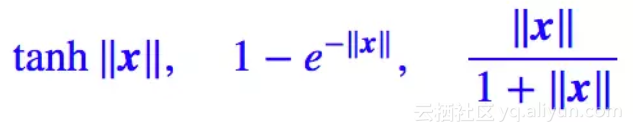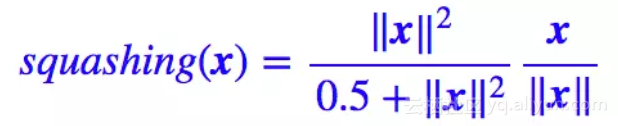y = tf.matmul(W, x) + b
y = tf.tanh(y)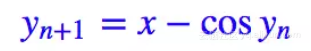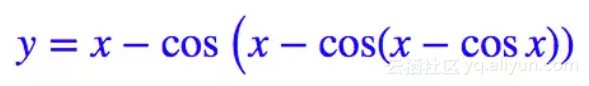y = tf.matmul(W, x) + b
Y=y
for i in range(3):
Y = y - tf.cos(Y)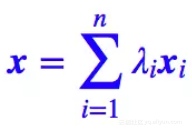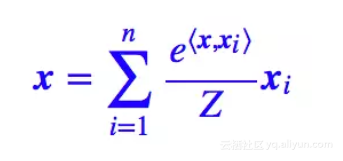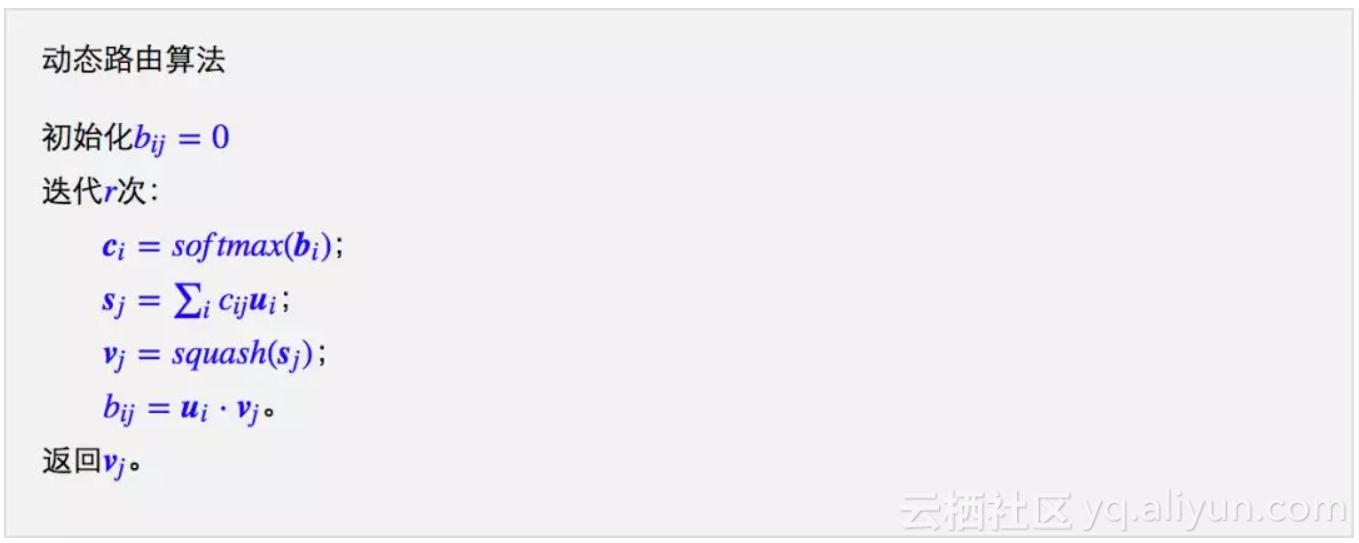“嘿，终于逮到个错误了，我看过论文，应该是 bij=bij+ui⋅vj 而不是 bij=ui⋅vj 吧”？

“难道是Hinton错了？就凭你也有资格向Hinton叫板”？别急别急，先让我慢慢分析 Hinton 的迭代出现了什么问题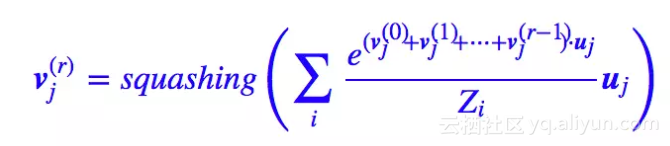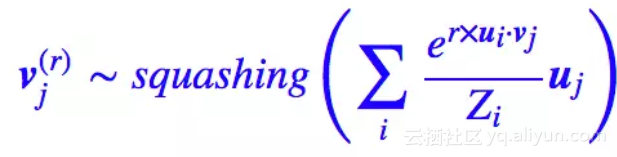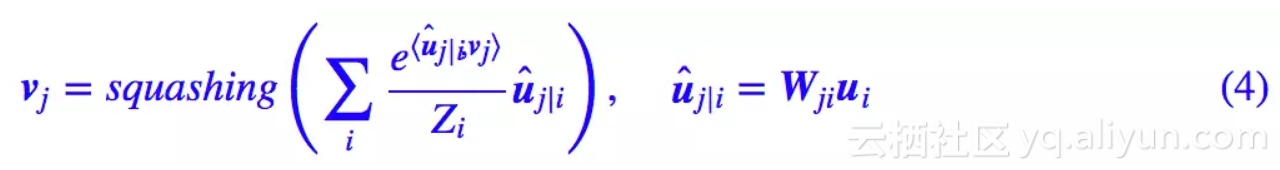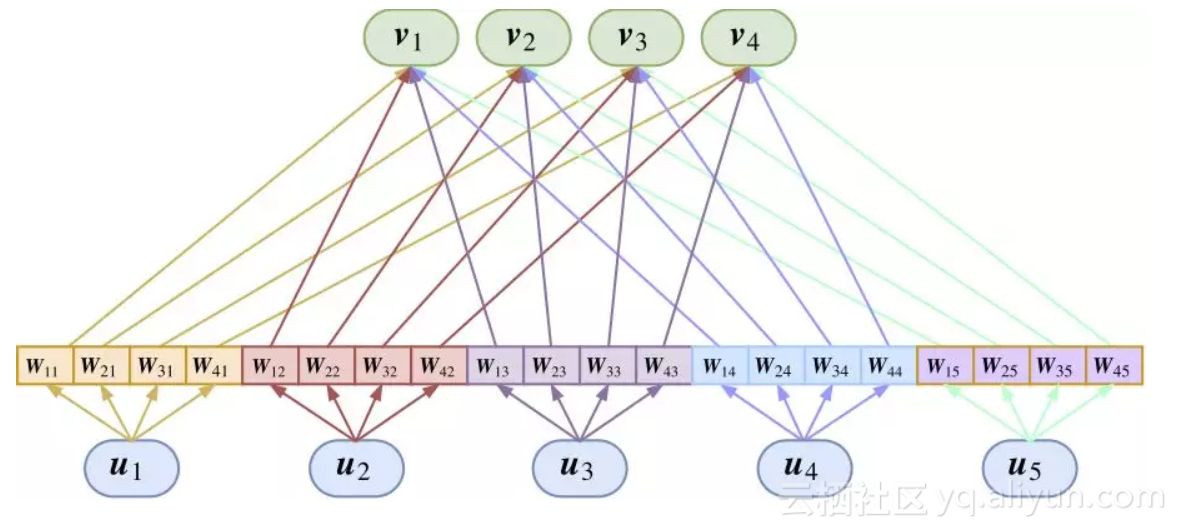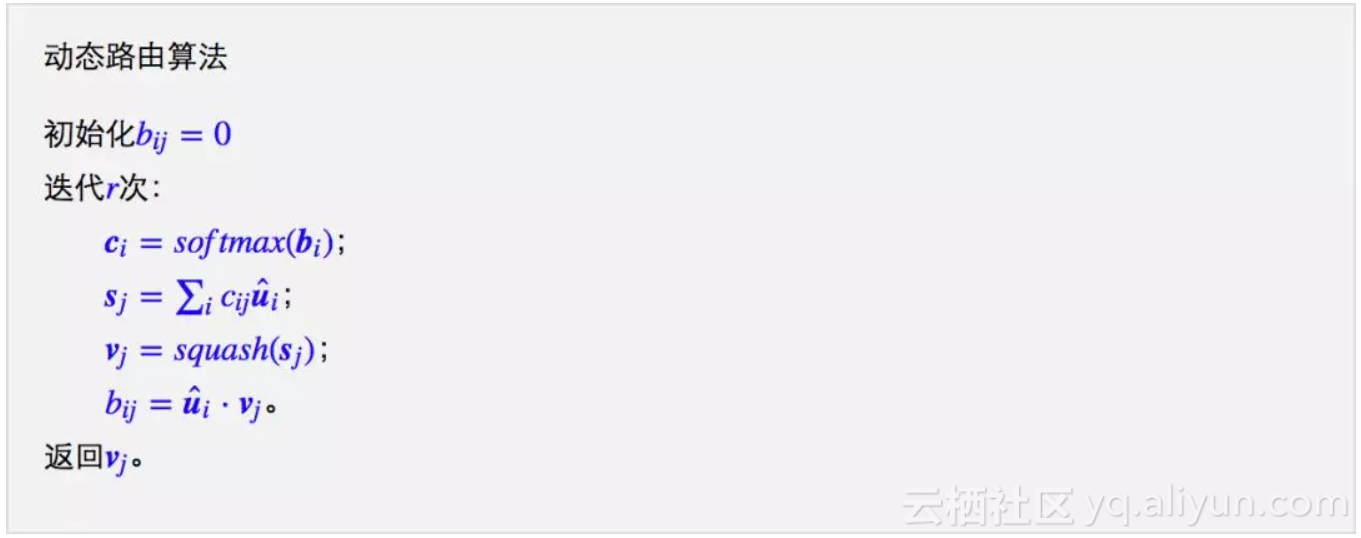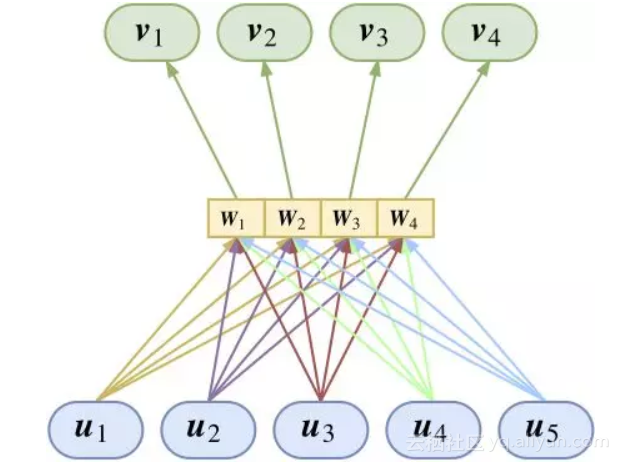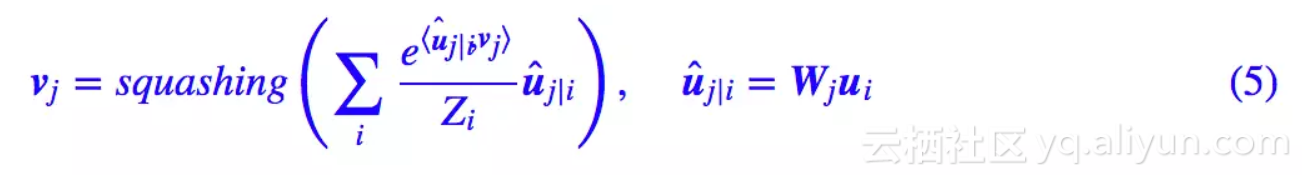MNIST 分类

Capsule 做分类模型，跟普通神经网络的一些区别是：Capsule 最后输出 10 个向量（也就是 10 个胶囊），这 10 个向量各代表一类，每个向量的模长代表着它的概率。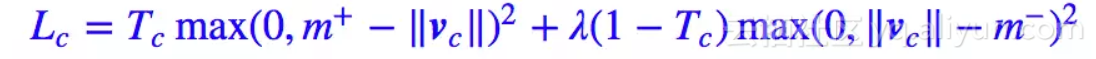1. 通过现有的 MNIST 数据集，训练一个数字识别模型，但最后不用 softmax 做 10 分类，而是转化为 10 个 2 分类问题；
2. 训练完模型后，用模型进行测试。测试的图片并不是原始的测试集，是随机挑两张测试集的图片拼在一起，然后看模型能不能预测出这两个数字来（数字对即可，不考虑顺序）。

Github: https://github.com/bojone/Capsule

#CNN部分，这部分两个模型都一致
input_image = Input(shape=(None,None,1))
cnn = Conv2D(64, (3, 3), activation='relu')(input_image)
cnn = Conv2D(64, (3, 3), activation='relu')(cnn)
cnn = AveragePooling2D((2,2))(cnn)
cnn = Conv2D(128, (3, 3), activation='relu')(cnn)
cnn = Conv2D(128, (3, 3), activation='relu')(cnn)

cnn = GlobalAveragePooling2D()(cnn)
dense = Dense(128, activation='relu')(cnn)
output = Dense(10, activation='sigmoid')(dense)

model = Model(inputs=input_image, outputs=output)
model.compile(loss=lambda y_true,y_pred: y_true*K.relu(0.9-y_pred)**2 + 0.25*(1-y_true)*K.relu(y_pred-0.1)**2,
metrics=['accuracy'])

#对测试集重新排序并拼接到原来测试集，就构成了新的测试集，每张图片有两个不同数字
idx = range(len(x_test))
np.random.shuffle(idx)
X_test = np.concatenate([x_test, x_test[idx]], 1)
Y_test = np.vstack([y_test.argmax(1), y_test[idx].argmax(1)]).T
X_test = X_test[Y_test[:,0] != Y_test[:,1]] #确保两个数字不一样
Y_test = Y_test[Y_test[:,0] != Y_test[:,1]]
Y_test.sort(axis=1) #排一下序，因为只比较集合，不比较顺序

Y_pred = model.predict(X_test) #用模型进行预测
greater = np.sort(Y_pred, axis=1)[:,-2] > 0.5 #判断预测结果是否大于0.5
Y_pred = Y_pred.argsort()[:,-2:] #取最高分数的两个类别
Y_pred.sort(axis=1) #排序，因为只比较集合

acc = 1.*(np.prod(Y_pred == Y_test, axis=1)).sum()/len(X_test)
print u'不考虑置信度的准确率为：%s'%acc
acc = 1.*(np.prod(Y_pred == Y_test, axis=1)*greater).sum()/len(X_test)
print u'考虑置信度的准确率为：%s'%acc

capsule = Capsule(10, 16, 3, True)(cnn)
output = Lambda(lambda x: K.sqrt(K.sum(K.square(x), 2)))(capsule)

model = Model(inputs=input_image, outputs=output)
model.compile(loss=lambda y_true,y_pred: y_true*K.relu(0.9-y_pred)**2 + 0.25*(1-y_true)*K.relu(y_pred-0.1)**2,
metrics=['accuracy'])

Capsule 致力于给出神经网络的可解释的方案，因此，从这个角度来看，Capsule 应该是成功的，至少作为测试版是很成功的。因为它的目标并不是准确率非常出众，而是对输入做一个优秀的、可解释的表征。

Hinton 想要抛弃反向传播的大概原因是：反向传播在生物中找不到对应的机制，因为反向传播需要精确地求导数。

loss 变小/大就意味着（近似的）梯度为负/正，这个过程的思想跟梯度下降是一致的，但这个过程一次性只能调节一个参数，而我们可能有数百万的参数要调，需要进行上百万次试验要才能完成每一个参数的调整。

Hinton 因为卷积中的池化是不科学的，但我并不这样认为。也许对于 MNIST 这个 28*28 的数据集并不需要池化也能 work，但如果是 1000*1000 的大图呢？越远的东西就越看不清，这难道不是池化的结果？

+ 关注